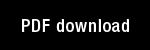Some nonlinear networks capable of learning a spatial pattern of arbitrary complexity

Author(s): Grossberg, S. |

Year: 1968

Citation: Proceedings of the National Academy of Sciences, 59 , 368-372

Abstract: Introduction: This note describes some nonlinear networks which caD learn a spatial pattern, in "black and white," of arbitrary size and complexity. These networks are a special case of a collection of learning machines ~ which were introduced in reference 1, where a machine capable of learxung a list of "letters" or "events" was described, We list in heuristic terminology some of the properties which arise in the learning of patterns:
(a) "Practice makes perfect": Given a "black and white" pattern of arbitrary size and complexity, a nonlinear network fit can be found vhich learns this pattern to any prescribed degree of accuracy.
(b) An isolated machine never forgets: If the pattern is leal'ned to a fixed degree of accuracy by 3Tl:, then fit will remember the pattern to at least this degree of accuracy until a new pattern is imposed upon fit.
(c) Overt practice is unnecessary: fit remembers the pattern without practicing it overtly,
(d) Contour enhancement: If fit learns the pattern to a "moderate" degree of accuracy, then fit's memory of the pa~tern spontaneously improves after practices ceases, As a result, when fit recalls the pattern, its contours are enhanced in the sense that "darks get darker" and "lights get lighter."
(e) A new pattern can always be learned: Even if 3Tl knows one pattern to an arbitrary degree of accuracy, this pattern can be replaced by any other pattern by a sufficient amount of practice.

Topics: Mathematical Foundations of Neural Networks, Models: Other,